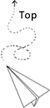## python 单例模式

• 使用模块
• 使用 new
• 使用装饰器（decorator）
• 使用元类（metaclass）

### 使用 new :

``````
class Singleton(object):
_instance = None

def __init__(self, a, *args, **kw):
self.a = a
print(self.a)

def __new__(cls, a, *args, **kw):
# NOTICE: __init__方法中除了self之外定义的参数，都将与__new__方法中除cls参数之外的参数是必须保持一致或者等效
if not cls._instance:
cls._instance = super(Singleton, cls).__new__(cls, *args, **kw)
return cls._instance

class NonSingleton(object):
def __init__(self, a, *args, **kw):
self.a = a
print(self.a)

class MySingleClass(Singleton):
a = 1

class MyNonSingleClass(NonSingleton):
a = 1

a1 = MySingleClass(1)
print(a1.a)
a2 = MySingleClass(2)
print(a1.a)
print(a2.a)

print(a1 is a2)  # --> True

a3 = MyNonSingleClass(3)
print(a3.a)
a4 = MyNonSingleClass(4)
print(a3.a)
print(a4.a)

print(a3 is a4)  # --> False
``````

More: